# How to create assignments

Quarterfall is all about creating and making assignments. Assignments can contain study material, like texts and videos, and one or more exercises. There are currently three types of exercises: multiple choice questions, open questions, and code questions. In this tutorial we will deal with multiple choice and open questions. In a separate tutorial we deal with code questions. One of the prime features of Quarterfall is to automatically create feedback for students. This will provide students with a much better learning experience, and it will save you as a teacher time. Feedback generation will also be demonstrated in this tutorial.

### What is an assignment?

In the first tutorial, you created your first assignment. Before we create some more, it is good to understand better what we mean with an assignment. Basically, an assignment is something that a student has to do. This can be reading a text or watching a video or presentation. But most of the time, it will involve answering questions.

Assignments are a crucial part of education. There are three different ways in which assignments are used: To help the students understand the learning material, to let them apply it, and to test whether they mastered the material. Quarterfall in particular focuses on the first: To give students a better understanding of the topics. This is often called formative assessment.

For formative assessment to be effective, it is important that students really try to make the assignments, and then get relevant feedback, such that they can see whether they understood the material or what mistakes they made. Below we will show how to achieve that.

### Create a course

As a first step, you need to create a course and a module in it. Assignments are always part of a module, and a module is always part of a course. We demonstrated this in the first tutorial, so have a look there if you are uncertain how to do this. We are going to create some assignments about the EU in this tutorial.

### Multiple choice

We will start with a simple multiple choice question. You already created one in the first tutorial, but here we will go a bit more into the details. Create a new assignment with the name "Establishment". Click Add Block  to add a new block and select the multiple choice question.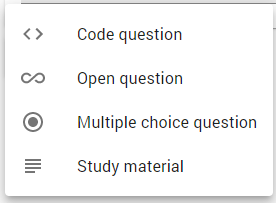As a first step we are going to fill in the question text. Our question is: "In what year was the EU established?". Next we need to create some choices for the student to pick from. For each choice, press Add Choice. We add the following choices: 1957, 1993, 2002, and 2009. Below it will become apparent why (if you don't already know). The correct answer is 1993. You should indicate this by selecting the radio button in front of it. So it will now look as follows: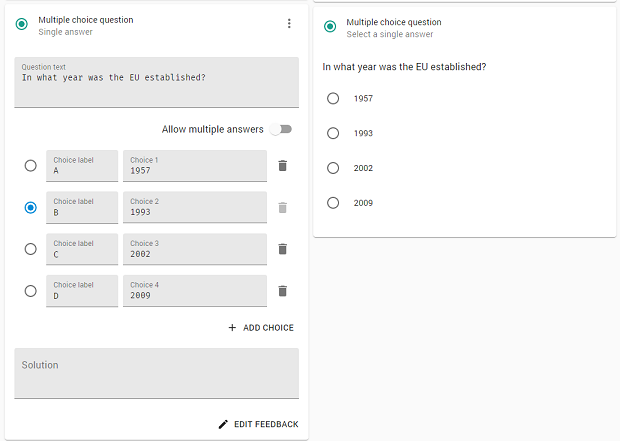At the right you will see a preview of the assignment, which you can also try out. When the student makes the question, he/she must complete the answer and is then shown the correct solution. You can also provide a more extensive solution yourself. For example, like this.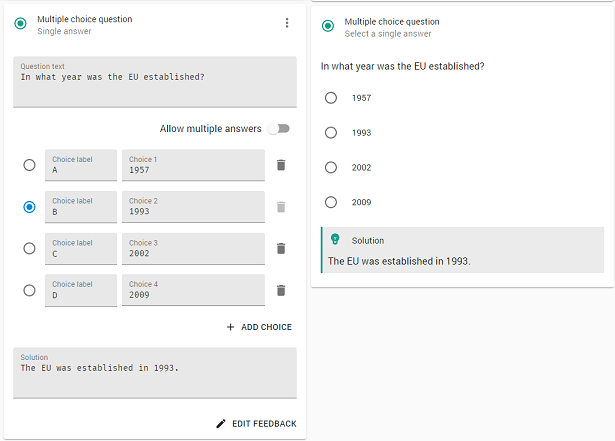The solution is now shown in the preview. Of course, the student will only see this solution after he/she completed the question.

#### Defining feedback

Although showing the solution is useful to the students, in particular when you provide a relevant solution text, it would be better if the feedback would depend on the answer of the student. So let us do that. But first, note that each choice you added has a field labeled Choice label. This is the name of the choice. We will need that to refer to the possible choices. You can change these to something more logical. But for the moment, let use keep the defaults.

Press the button Edit Feedback to go to the feedback editor. This will look as follows: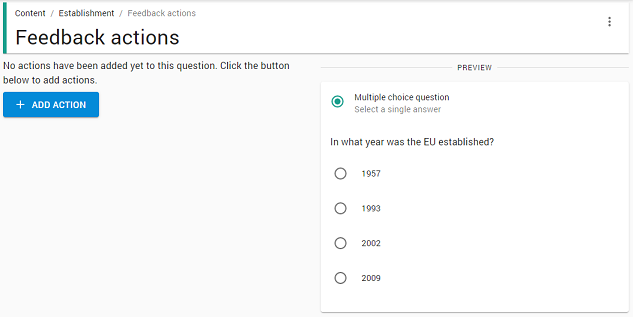At the left there is a, currently empty, list of actions. At the right there is a preview of the multiple choice question, such that you an easily test whether the feedback works correctly. Press Add Action to show the following menu: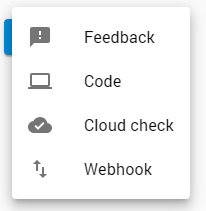Here we will only use the first option: Feedback, so select that one. For the others, see the documentation of feedback.  You will now be shown a feedback action: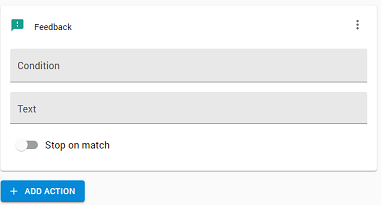A feedback action has a condition and a feedback text. The feedback text is shown to the student when the condition is satisfied (true). In the condition you can refer to the answer of the student, and you can use the choice labels. So, for example, you can use condition answer == "A" and as feedback text: "No, that is not correct. In 1957 the ECC was founded, which was the predecessor of the EU." Note that you must use == for "is equal", not a single =. Also, note that the label must be put between quotes if used the check whether it is the answer.

Try it out by selecting 1957 in the preview at the right, and press the button Check Answer. You (like the student) will see the following: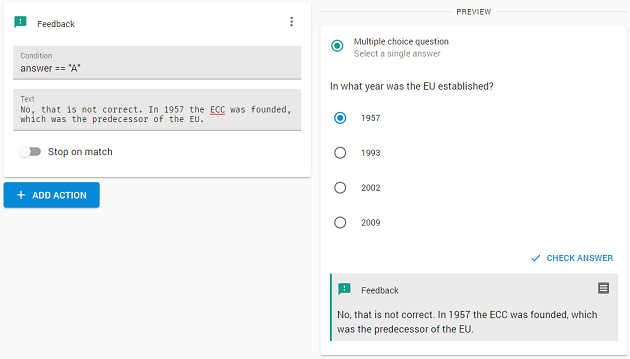Note the log icon at the top-right of the feedback. If something goes wrong you can inspect this log to help you debug the actions. (This is not shown to the students.)

Now you can continue to add three more feedback actions for the other three possible choices, using the  Add Action button again. For 1993 you should indicate the answer is correct, for 2002 you should indicate that that was the year the Euro was introduced, and for 2009, that that was the year of the Lisbon treaty that changed the rules in the EU considerably.

There is a lot more to know about generating feedback. First of all, the labels themselves can actually be used in the conditions. So instead of answer == "A" you could just have written A. (It is a variable now, so no quotes!). Also, as you will have seen, there is a switch called Stop on match. If you switch this on, and the condition is true, no further actions will be executed. This can help keeping your conditions simple. And there are also a lot more things you can do in the conditions, instead of checking the answer. We will use this in the examples below.

In the question above, only one answer was correct. But you can also allow for multiple answers. This is very simple, just use the switch Allow for multiple answers. The round radio buttons are now replaced by square check marks, to indicate multiple answers are possible. For example, we can create a question about member states of the EU, as follows: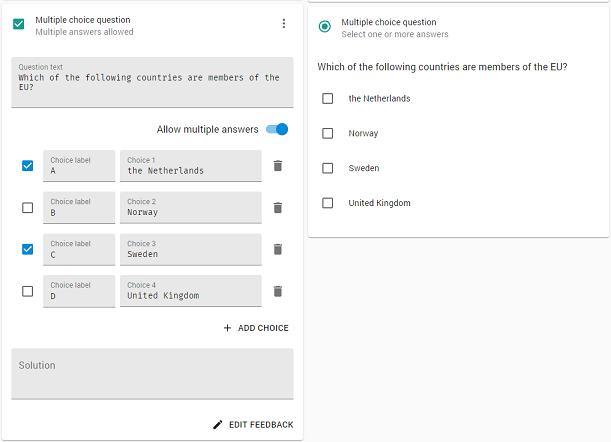Note that the UK is no longer a member of the EU! Defining feedback is now a bit more difficult, as there are many possible combinations of choices. Fortunately, the conditions in feedback actions can handle this pretty easily. First of all, a condition is actually a Boolean expression. Boolean expressions are built up as follows:

• Use == for equal. Also you can use comparisons like <, <=, > and >=.
• Use && for and, || for or, and ! for not.
• You can also use parentheses.

A condition is actually a JavaScript expression that must have as a value true or false. You can use all functionality of JavaScript to create your conditions. You you can e.g. check for correctness of the answer using: A && !B && C && !D. But there is actually also a variable correct, that indicates whether the answer is correct. So your first feedback action could look as follows: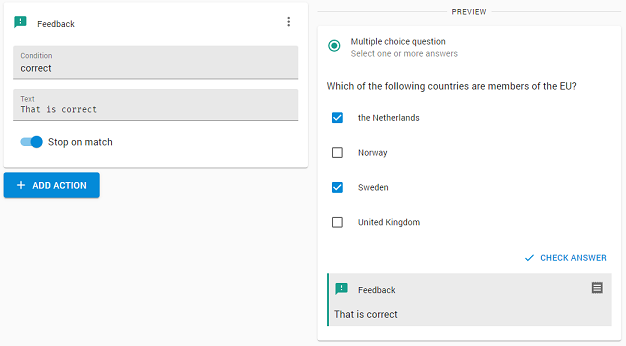Note the use of Stop on match. As the further feedback actions deal with mistakes we do not want to execute them when the answer is correct. The next feedback action should now indicate that the answer is wrong. So add an action, leave the condition blank (it is always executed then), and as a text write "That is not correct."

You could leave it there, but that does not give much help to the student. So you might e.g. add the following actions:

• B: Norway has never been a member of the EU.
• D: Since early 2020 the United Kingdom in no longer part of the EU.
• !A || !C: There are more member countries than you indicated.

Note that multiple of these conditions can be true, so multiple feedback texts can be shown.

### Open questions

In an open question, the students can type any answers they like. This obviously will make it harder to automatically generate relevant feedback. But you can still do some useful tests.

It is possible to restrict the answer to being a number, which can obviously be tested easily. As an example, let us add a question about the number of member states. Create a new assignment. Press the Add Block button and choose on Open Question. As text, use something like "How many member states has the EU?". As answer field type, select Number. So your assignment looks as follows: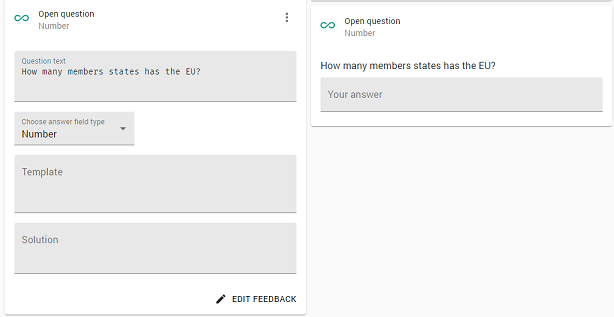You can provide a solution, but as we want to give more relevant feedback, we won't be using this. You can also provide a template for the answer. We will discuss templates in the tutorial on code questions. Press Edit Feedback to define feedback for this question.

As before, we will first handle the correct case. In this case, the answer should be 27, so add a feedback action with condition answer == 27, as follows: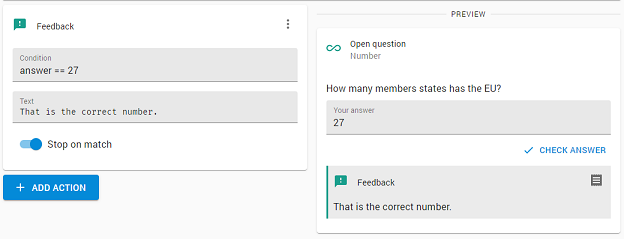Note that we switched Stop on match on. Now we can add some more cases:

• answer == 28: Almost correct. Since early 2020 the UK is no longer part of the EU.
• answer <= 24: No, the EU is considerably larger.
• answer >= 30: The commission would probably like this, but the EU is not that large.

In all cases, switch Stop on match on, to make sure just one of the feedback texts is displayed.

When the answer to an open question can be any text, checking it automatically is a lot harder. But by formulating the question carefully and looking for keywords, you can still provide useful feedback. Here is an example.

Create a new assignment and add an Open question block. We keep the field type to text. As a question use: "What important treaties where signed by the EU members in 1993 and 2009?". Now, obviously, we don't know exactly what the student is going to type in. But the correct answer should contain the words Maastricht and Lisbon, as those are the names of the two treaties. So we should check whether the answer contains those words.

Although we can do such checks directly inside the feedback conditions, we are going to use a slightly different approach here. Click on Edit Feedback and click on Add Action, but this time choose a Code action. A little code editor appears in which you can type a piece of JavaScript code. Here you can do all sorts of computation based on the answer of the student. The answer of the student is stored in  qf.answer. qf is an object that contains as members all the variables that you can use in conditions. You can also add new members that you can then use in later feedback conditions. We will check for the presence of the words Maastricht and Lisbon and store the results, as follows: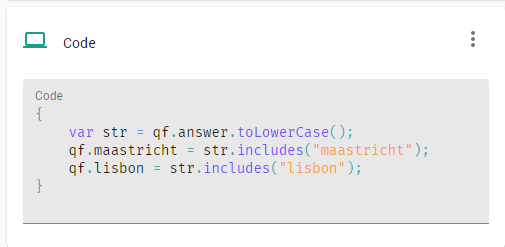So we created two additional variables that we can now use in the feedback actions. So we create three feedback actions:

• maastricht && lisbon: Indeed Maastricht and Lisbon are the two treaties.
• !maastricht: In 1993 the members signed the Maastricht treaty, with which the EU was founded.
• !lisbon: In 2009 the members signed the Lisbon treaty, that changed the rules in the EU.

You could also add a check for Rome, as that is a possible mistake, as the ECC was founded at the treaty.

Using code action you can do a lot more analysis of the answer. And if that is not enough, you can even send the answer to external web hooks to do further processing.

### Well done

Now you should know enough to make lots of useful assignment for your student and define relevant feedback for them. So it is time to add some students to allow them to make your assignments.